Custom SearchCOMPOUND PROBABILITIES The probabilities to this point have been single events. In the discussion on compound probabilities, events that may affect others will be covered. The word may is used because independent events are included with dependent events and mutually exclusive events. INDEPENDENT EVENTS Two or more events are independent if the occurrence or nonoccurrence of one of the events has no affect on the probability of occurrence of any of the others. When two coins are tossed at the same time or one after the other, whether one falls heads or tails has no affect on the way the second coin falls. Suppose we call the coins A and B. The coins may fall in the following four ways: 1. A and B may fall heads. 2. A and B may fall tails. 3. A may fall heads and B may fall tails. 4. A may fall tails and B may fall heads. The probability of any one way for the coins to fall is calculated as follows: s=4 and n=4 therefore,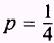This probability may be determined by considering the product of the separate probabilities; that is, the probability that A will fall heads isthe probability that B will fall heads is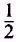and the probability that both will fall heads is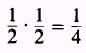In other words, when two events are independent, the probability that one and then the other will occur is the product of their separate probabilities. EXAMPLE: A box contains 3 red marbles and 7 green marbles. If a marble is drawn, then replaced, and another marble is drawn, what is the probability that both marbles are red? SOLUTION: Two solutions are offered. First, by the principle of choice, 2 marbles can be selected in 10 . 10 ways. The red marble may be selected on the first draw in three ways and on the second draw in three ways; and by the principle of choice, a red marble may be drawn on both trials in 3 . 3 ways. Then the required probability isThe second solution, using the product of independent events, follows: The probability of drawing a red marble on the first draw is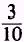, and the probability of drawing a red marble on the second draw is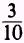. Therefore, the probability of drawing a red marble on both draws is the product of the separate probabilities orPRACTICE PROBLEMS: 1. If a die is tossed twice, what is the probability of rolling a 2 followed by a 3? 2. A box contains 2 white, 3 red, and 4 blue marbles. If after each selection the marble is replaced, what is the probability of drawing, in order a. a white then a blue marble? b. a blue then a red marble? c. a white, a red, then a blue marble? ANSWERS:Integrated Publishing, Inc. - A (SDVOSB) Service Disabled Veteran Owned Small Business